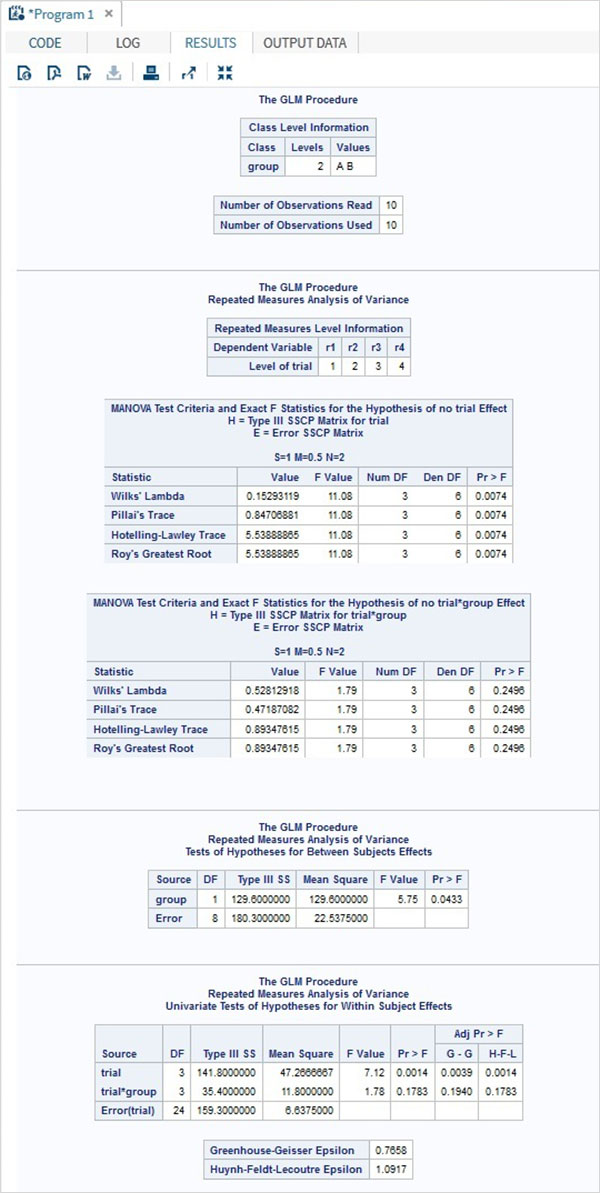# SAS - Repeated Measure Analysis

Repeated measure analysis is used when all members of a random sample are measured under a number of different conditions. As the sample is exposed to each condition in turn, the measurement of the dependent variable is repeated. Using a standard ANOVA in this case is not appropriate because it fails to model the correlation between the repeated measures.

One should be clear about the difference between a repeated measures design and a simple multivariate design. For both, sample members are measured on several occasions, or trials, but in the repeated measures design, each trial represents the measurement of the same characteristic under a different condition.

In SAS PROC GLM is used to carry out repeated measure analysis.

## Syntax

The basic syntax for PROC GLM in SAS is −

```PROC GLM DATA = dataset;
CLASS variable;
MODEL variables = group / NOUNI;
REPEATED TRIAL n;
```

Following is the description of the parameters used −

• dataset is the name of the dataset.

• CLASS gives the variables the variable used as classification variable.

• MODEL defines the model to be fit using certain variables form the dataset.

• REPEATED defines the number of repeated measures of each group to test the hypothesis.

## Example

Consider the example below in which we have two groups of people subjected to test of effect of a drug. The reaction time of each person is recorded for each of the four drug types tested. Here 5 trials are done for each group of people to see the strength of correlation between the effect of the four drug types.

```DATA temp;
INPUT person group \$ r1 r2 r3 r4;
CARDS;
1 A  2  1  6  5
2 A  5  4 11  9
3 A  6 14 12 10
4 A  2  4  5  8
5 A  0  5 10  9
6 B  9 11 16 13
7 B  12 4 13 14
8 B  15 9 13  8
9 B  6  8 12  5
10 B 5  7 11  9
;
RUN;

PROC PRINT DATA = temp ;
RUN;

PROC GLM DATA = temp;
CLASS group;
MODEL r1-r4 = group / NOUNI ;
REPEATED trial 5;
RUN;
```

When the above code is executed, we get the following result −